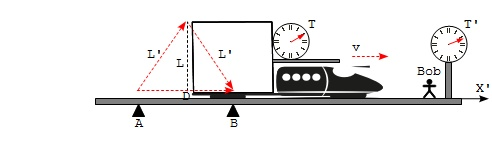Demonstration of Time Dilation - Formula

This section continues the thought experiment to demonstrate time dilation. An isosceles triangle is used to derive the time dilation formula with Lorentz factor.

Part 3 - Time Dilation Formula: The third part of the thought experiment is to derive the time dilation formula using the Pythagorean theorem. This part consists of the following:

1. Establish an isosceles triangle with trajectories of the laser meter and light pulse in Bob's frame:

• The trajectory of the laser meter forms the base of the isosceles triangle, D.
• The trajectory of the light pulse forms two legs of the isosceles triangle, L' and L'.

2. Establish a relation between the leg length L and L' based on the Pythagorean theorem:

```L'**2 = D**2 + L**2           (T.9) - Pythagorean theorem
2*D = v*T'                   (T.10) - maser meter moves at speed v
L'**2 = (v*T'/2)**2 + L**2   (T.11) - merging T.10 into T.9
```

3. Calculate time dilation factor:

```2*L = c*T                     (T.1) - Amy's observation
2*L' = c*T'                   (T.3) - Bob's observation

L'**2 = (v*T'/2)**2 + (c*T/2)**2       (T.12) - merging T.1 into T.11
4*L'**2 = (v*T')**2 + (c*T)**2         (T.13) - multiplying by 4
4*(c*T'/2)**2 = (v*T')**2 + (c*T)**2   (T.14) - merging T.3 into T.13
(c*T')**2 = (v*T')**2 + (c*T)**2       (T.15) - simplifying left side
(c*T')**2 - (v*T')**2 = (c*T)**2       (T.16) - moving T' terms together
(c**2-v**2)*T'**2 = (c*T)**2           (T.17) - factoring T'

sqrt(c**2-v**2)*T' = c*T     (T.18) - taking square root
T' = (c/sqrt(c**2-v**2))*T   (T.19) - normalizing on T'
T' = (1/sqrt(1-(v/c)**2))*T  (T.20) - moving c into sqrt()
# Time dilation formula
```

Voila! We derived the dilation formula. Bob's observed that Amy's bouncing light pulse clock is slower by a factor of (1/sqrt(1-(v/c)**2)), which is called Lorentz Factor (named after Hendrik Lorentz).Isosceles Triangle to Calculate Time Dilation